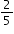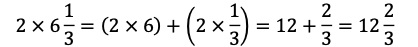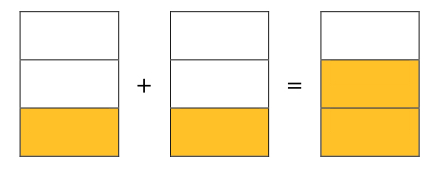# MA.4.AR.1.3Export Print
Solve real-world problems involving multiplication of a fraction by a whole number or a whole number by a fraction.

### Examples

Ken is filling his garden containers with a cup that holdspounds of soil. If he uses 8 cups to fill his garden containers, how many pounds of soil did Ken use?

### Clarifications

Clarification 1: Problems include creating real-world situations based on an equation or representing a real-world problem with a visual model or equation.

Clarification 2: Fractions within problems must reference the same whole.

Clarification 3: Within this benchmark, the expectation is not to simplify or use lowest terms.

Clarification 4: Fractions limited to fractions less than one with denominators of 2, 3, 4, 5, 6, 8, 10, 12, 16 and 100.

General Information
Subject Area: Mathematics (B.E.S.T.)
Strand: Algebraic Reasoning
Status: State Board Approved

## Benchmark Instructional Guide

• Equation
• Expression
• Whole Number

### Vertical Alignment

Previous Benchmarks

Next Benchmarks

### Purpose and Instructional Strategies

The purpose of this benchmark is to complement the instruction of MA.4.FR.2.4 with real-world context.
• Instruction should refer back to the two types of problems described in MA.4.FR.2.4 (whole number times a fraction and fraction times a whole number), and give students opportunities to work with real-world examples of both types.
• Instruction should help students bring their understanding of working with units involving whole numbers (1 cup, 2 miles, etc.) to working with units involving fractions ($\frac{\text{1}}{\text{2}}$ cup, $\frac{\text{3}}{\text{4}}$ miles, etc.)
• During instruction it is acceptable to have students work with problems where  fractional parts represent more than 1 whole (e.g., a cake recipe calls for $\frac{\text{1}}{\text{3}}$ cup of water  and $\frac{\text{1}}{\text{8}}$ oz of vanilla extract, and the baker wants to double the recipe).
• Instruction may include having students create real-world situations that can be modeled by a given expression like $\frac{\text{3}}{\text{5}}$× 10 (I have completed $\frac{\text{3}}{\text{5}}$ of my 10-mile run).
• During instruction, models and explanations should relate fraction multiplication to equal groups. This will activate prior knowledge and relate what students know to whole-number multiplication.
• For example, teachers can help students make connections to multiplication from grade 3 by referring to an expression like 4 × $\frac{\text{3}}{\text{5}}$ as “ four groups of 3 fifths,” that 5 is, “ four groups that each contain 3 items and each item is one fifth” (MTR.2.1, MTR.5.1).
• Example: have table/bar to show that there are 4 groups of $\frac{\text{3}}{\text{5}}$
• Exploring patterns of what happens to the numerator when a whole number is multiplied by a fraction will help students make sense of multiplying fractions by fractions in grade 5 (MTR.2.1). When multiplying whole numbers by mixed numbers, students can use the distributive property or write the mixed number as a fraction greater than one. During instruction, students should compare both strategies (MTR.6.1). Using the distributive property to multiply a whole number by a mixed number could look like this.• In the example, 2 groups of 6 $\frac{\text{1}}{\text{3}}$ was written as “ the sum of 2 groups of 6 and 2 groups of 1 third.” The products of 12 and 2 thirds are added to show the product of 12 $\frac{\text{2}}{\text{3}}$.

### Common Misconceptions or Errors

• Students may not understand that fractions are numbers (just as whole numbers are numbers) and this misconception may at first be reinforced by the fact that the phrase “ group size” works well with whole numbers, but not so well with fractions; therefore, special attention should be given with many different real-world examples.
• Students may not understand what a fractional portion represents within the context of a real-world situation.

### Strategies to Support Tiered Instruction

• Instruction includes opportunities to engage in teacher-directed practice using visual representations to solve real-world problems involving the multiplication of a fraction by a whole number. Students are directed on how to use models or equations based on real-world situations. Through questioning, the teacher guides students to explain what each fractional portion represents in the problems used during instruction and practice.
• For example, the teacher displays and reads aloud the following problem: “ Angelica walks her dog $\frac{\text{4}}{\text{5}}$ of a mile every day. How far does she walk her dog after 7 days?” Using models, students solve the problem with explicit instruction and guided questioning. Students explain how to use models to solve this addition by guiding students to represent this problem as $\frac{\text{4}}{\text{5}}$ + $\frac{\text{4}}{\text{5}}$ + $\frac{\text{4}}{\text{5}}$ + $\frac{\text{4}}{\text{5}}$ + $\frac{\text{4}}{\text{5}}$ + $\frac{\text{4}}{\text{5}}$ + $\frac{\text{4}}{\text{5}}$ and that 7 × $\frac{\text{4}}{\text{5}}$ is the same as seven groups of four fifths. The teacher guides students to create an equation to represent the problem, repeating multiple real-world problems that involve the multiplication of a whole number by a fraction or a fraction by a whole number.
• Instruction includes opportunities for students to use hands-on models and manipulatives to solve real-world problems involving the multiplication of a whole number by a fraction. Students are guided in explaining how each model represents the real-world situation. Explicitly direct students on how to use models or equations based on real-world situations. Through questioning, students explain what each fractional portion represents in the problems used during instruction and practice.
• For example, the teacher displays and reads aloud the following problem: “ Ramon is baking cupcakes for his cousin’s birthday party. The recipe calls for 2 cups of sugar. He only needs to make $\frac{\text{1}}{\text{3}}$ as many cupcakes as the recipe call for. How many cups of sugar will Ramon need to use?” It may be useful to provide students with paper models that represent the cups of sugar. Students should cut the models into thirds to determine how much sugar will be needed for the recipe.Lorelei is having a dessert party and wants to determine how much sugar she will need. For the party, she will make 4 batches of chocolate chip cookies and 8 vanilla smoothies. 1 batch of chocolate chip cookies requires $\frac{\text{2}}{\text{3}}$ cup of sugar and 1 vanilla smoothie requires $\frac{\text{1}}{\text{3}}$ cup of sugar. How much total sugar will she need for her dessert party? Draw a model to explain your thinking.

### Instructional Items

Instructional Item 1

A butcher has 10 pounds of meat and sells $\frac{\text{2}}{\text{3}}$ of it in one day. How many pounds does the butcher sell?

*The strategies, tasks and items included in the B1G-M are examples and should not be considered comprehensive.

## Related Courses

This benchmark is part of these courses.
5012060: Mathematics - Grade Four (Specifically in versions: 2014 - 2015, 2015 - 2022, 2022 and beyond (current))
7712050: Access Mathematics Grade 4 (Specifically in versions: 2014 - 2015, 2015 - 2018, 2018 - 2022, 2022 and beyond (current))
5012065: Grade 4 Accelerated Mathematics (Specifically in versions: 2019 - 2022, 2022 and beyond (current))
5012015: Foundational Skills in Mathematics 3-5 (Specifically in versions: 2019 - 2022, 2022 and beyond (current))

## Related Access Points

Alternate version of this benchmark for students with significant cognitive disabilities.
MA.4.AR.1.AP.3: Solve one-step real-world problems involving multiplication of a unit fraction by a whole number (e.g., 3 ×, 2 ×, 5 ×). Denominators limited to 2, 3, 4, 6, 8 or 10.

## Related Resources

Vetted resources educators can use to teach the concepts and skills in this benchmark.

## Lesson Plans

Lessen the Litter:

Students will calculate the total amount of trash at different locations in the community to determine which location has the most trash and explore ways a community can work together to prevent future trash buildup in this integrated lesson plan.

Type: Lesson Plan

Modeling Multiple Groups of Fractions:

In this inquiry lesson students will use a situational story to explore ways to find the total quantity of a fraction multiplied by a whole number using various models.

Type: Lesson Plan

Multiple Bake Sale Cookie Recipes with fractional ingredients:

In this lesson students will explore ways to find the product of mixed numbers multiplied by a whole number using a real-world situation.

Type: Lesson Plan

Modeling Multiplication with Fractions:

Students will relate multiplication strategies with fractions through problem solving situations. This lesson connects prior understanding of multiplication and equal groups to multiplication of fractions.

Type: Lesson Plan

Multiple Bake Sale Cookie Recipes with fractional ingredients PART 1:

In this lesson students are guided through the process of multiplying a whole number and a fraction in a real-world situation.  The lesson uses the number line to explain the process.

Type: Lesson Plan

Multiply Fractions and Whole Numbers with Models:

Students will multiply a whole number by a fraction through set models and problem solving.

Type: Lesson Plan

## Original Student Tutorial

Dream House Dilemma Part 1: Area:

Help April calculate area and missing measurements for items in her perfect dream home in this interactive tutorial.

This is the first in a three-part series.  Click below to open the other tutorials in this series

Type: Original Student Tutorial

Sugar in six cans of soda:

This task provides a familiar context allowing students to visualize multiplication of a fraction by a whole number. This task could form part of a very rich activity which includes studying soda can labels.

## Original Student Tutorials Mathematics - Grades K-5

Dream House Dilemma Part 1: Area:

Help April calculate area and missing measurements for items in her perfect dream home in this interactive tutorial.

This is the first in a three-part series.  Click below to open the other tutorials in this series

## Student Resources

Vetted resources students can use to learn the concepts and skills in this benchmark.

## Original Student Tutorial

Dream House Dilemma Part 1: Area:

Help April calculate area and missing measurements for items in her perfect dream home in this interactive tutorial.

This is the first in a three-part series.  Click below to open the other tutorials in this series

Type: Original Student Tutorial

Sugar in six cans of soda:

This task provides a familiar context allowing students to visualize multiplication of a fraction by a whole number. This task could form part of a very rich activity which includes studying soda can labels.